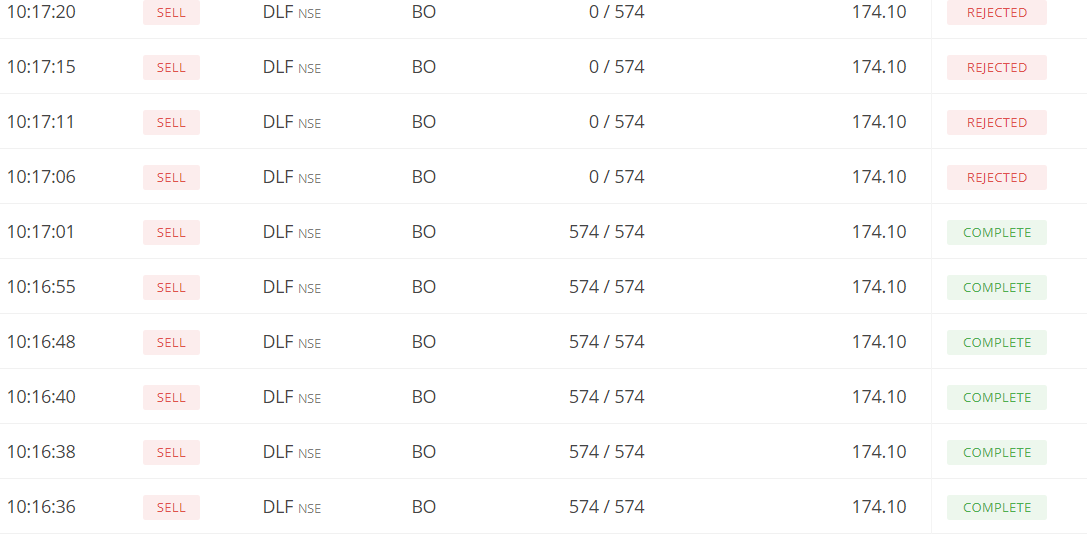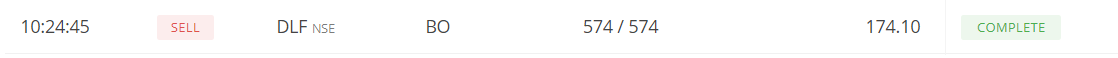# Multiple orders are getting created when I placed only one order using KITE API

Hello,

As per my code, the program would exit after placing the order. My program exited after placing the order for DLF (574 shares) for the LIMIT price of Rs.174.1 as expected

` result = kite.place_order(variety='bo', order_type='LIMIT', product='MIS', exchange='NSE', transaction_type='SELL',tradingsymbol=trading_symbol, price=sell_price, stoploss=stop_loss_point, quantity=quantity, squareoff=target_point) print(result) exit(0)`

But, when I logged into my kite account, I noticed multiple orders (7 orders) were created @ 10.16 AM. And new orders were being created continuously but was rejected only because no margin available.After 10 mins at 10.24 AM, when DLF hit the price of 174.1, one more order created automatically though my program exited long beforeTagged:
• The Kite Connect libraries are tested and are being used in many production systems including our apps. I would recommend checking your implementation.
• Thanks Sujith for the quick update. I'll check.
• edited May 2019
I could not figure out what is wrong in the following small piece of code. From the log, place_order is executed only once but order was placed twice today as well. Any help is appreciated.

``orderPlaced = Falsewhile(True):    if orderPlaced == True:        break    try:        print('inside while')        time.sleep(4)        results = kite.ltp(names)        print(results)        for index, row in df.iterrows():            if (results[row['NAME']]['last_price']) >= df.at[index, 'BUY_PRICE'] and \                    row['NAME'] not in blacklist:                trading_symbol = row['NAME'].split(':')                buy_price = row['BUY_PRICE']                target_point = tick_price(round_up(((buy_price / 100) * target) , 2 ))                stop_loss_point = tick_price(round_up(((buy_price / 100) * stoploss) ,2 ))                quantity = int(investment/buy_price)                try:                    print(f"BUY ORDER PLACED FOR : {trading_symbol}")                    orderPlaced = True                    blacklist.append(row['NAME'])                    result = kite.place_order(variety='bo', order_type='LIMIT', product='MIS', exchange='NSE',                                             transaction_type='BUY',tradingsymbol=trading_symbol, price=buy_price, stoploss=stop_loss_point, quantity=quantity, squareoff=target_point)                    print(result)                    orderPlaced = True                    break                except:                    print("Exception occured while placing buy order")                    exit(0)            elif (results[row['NAME']]['last_price']) <= df.at[index,'SHORT_PRICE'] and \                    row['NAME'] not in blacklist:                trading_symbol = row['NAME'].split(':')                sell_price = row['SHORT_PRICE']                target_point = tick_price(round_up(((sell_price / 100) * target) , 2 ))                stop_loss_point = tick_price(round_up(((sell_price / 100) * stoploss) ,2 ))                quantity = int(investment / sell_price)                try:                    print(f"SHORT ORDER PLACED FOR : {trading_symbol}")                    orderPlaced = True                    blacklist.append(row['NAME'])                    result = kite.place_order(variety='bo', order_type='LIMIT', product='MIS', exchange='NSE',                                              transaction_type='SELL',tradingsymbol=trading_symbol, price=sell_price, stoploss=stop_loss_point, quantity=quantity, squareoff=target_point)                    print(result)                    break                except:                    print("Exception occured while placing sell order")                    exit(0)    except:        pass``

Logs:
SHORT ORDER PLACED FOR : TATAMOTORS
190509000818083
Process finished with exit code 0

• @sathik
• Use round(value, 1) so that the price are rounded to the nearest one-tenth. 100.12 is incorrect price, where round(100.12, 1), which is 100.1, is correct.

• Bracket orders are multi-legged. There will be more than one orders. Can you show your open (or trigger pending) orders?
• @vijoeyz
• No problem with rounded values as I get the price ticks such as 100.05 / 100.10 / 100.15 only. Also, the system will not accept the value such as 100.12 and BO order will not be placed at all in the first place.
• All my orders were executed so I did not have any open orders. When you say bracket orders are multi-legged, what I understand is that when the order gets executed, two more orders will be placed, one for profit-taking and another for stop loss. The problem what I'm facing is order itself getting placed multiple times repeatedly.
There are two issues:
1. How multiple orders are placed by calling place_order() just once. I verified with my logs that the program exited as soon as the order was placed. I tried again by placing more traces and confirmed that place_order() was executed just once.
2. The second issue as I pointed with my order page the screen shot above is, even after the program terminated after about 10 mins, when the the same price triggered for which I placed my initial BO order, orders are executed again. It seems like whenever that price is triggered, the order will be placed throughout the day (as per the behavior)
• @sathik
How multiple orders are placed by calling place_order() just once.
From above code,it can only happen if an exception occurs before going inside try except block and main try block is repeated again, causing place_order to be executed twice.Can you DM me your complete code block including values of df data,tick_price method, etc.So,we can test the same at our end.